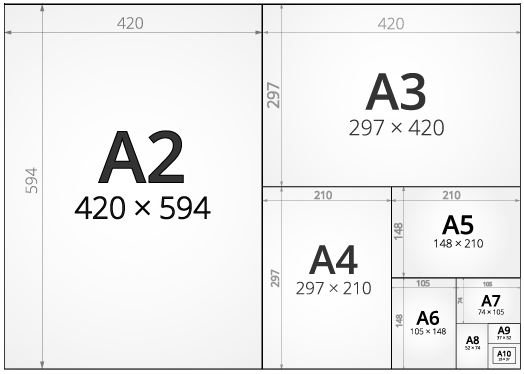﻿ A2 in Millimeters

# A2 paper size in millimeters (a2 in mm)

The dimensions of A2 paper are 594 x 420 millimeters. Choose any paper size and any unit length to show its dimensions.

 A5 paper size A4 paper size A3 paper size A2 paper size A1 paper size A0 paper size B5 paper size B4 paper size B3 paper size B2 paper size B1 paper size B0 paper size in millimeters inches centimeters =

Choose paper size and unit length### Dimensions of papers

 A5 in millimeters A5 in inches A5 in centimeters A4 in millimeters A4 in inches A4 in centimeters A3 in millimeters A3 in inches A3 in centimeters A2 in millimeters A2 in inches A2 in centimeters A1 in millimeters A1 in inches A1 in centimeters A0 in millimeters A0 in inches A0 in centimeters B5 in millimeters B5 in inches B5 in centimeters B4 in millimeters B4 in inches B4 in centimeters B3 in millimeters B3 in inches B3 in centimeters B2 in millimeters B2 in inches B2 in centimeters B1 in millimeters B1 in inches B1 in centimeters B0 in millimeters B0 in inches B0 in centimeters